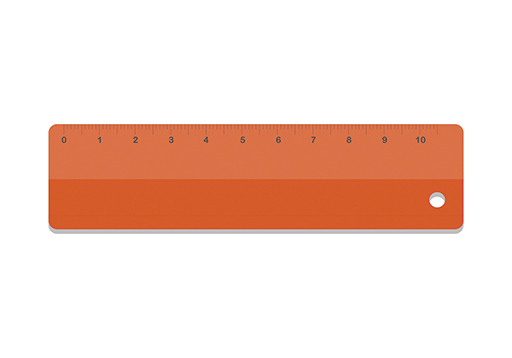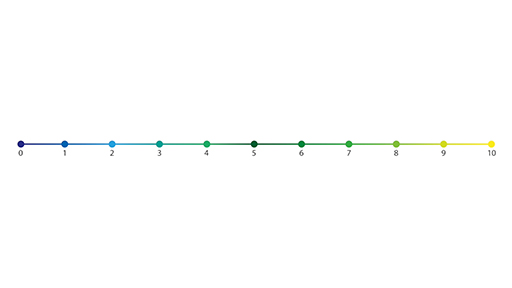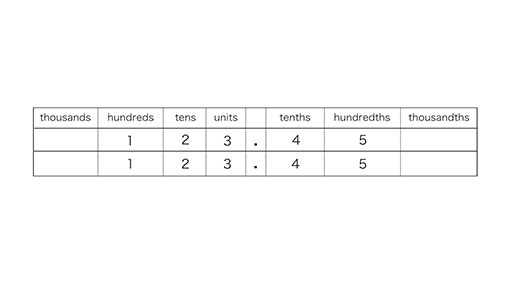Teaching mathematics

Start this free course now. Just create an account and sign in. Enrol and complete the course for a free statement of participation or digital badge if available.

Free course

# 4.2 Becoming familiar with decimals

Adding and subtracting decimals can be done using the same methods as adding and subtracting whole numbers as long as the digits are lined up under the correct column headings, keeping the decimal points underneath each other.

Learners can struggle to handle decimals, so it is helpful to use real-life instances with which the learners are familiar.

Numbers with one decimal place are evident on a classroom ruler set out in centimetres (Figure 6).Figure 6 A ruler showing centimetres and tenths of centimetres

There are 10 gradations between each whole number (of centimetres), so each interval is 1 tenth or 0.1. Rulers are effective number lines which learners have easy access to.

A useful activity to use with learners who struggle with decimals is to ask them to draw a straight line across a page. Next, they should put a mark near the beginning and label it A. Ask them to put another mark 4.2 centimetres along the line from A and to label it B. Next ask them to put another mark 2.9 centimetres along the line from B and label it C. Now ask them to measure from A to C. Then ask how they can check their answer (i.e. add up 4.2 and 2.9). This activity (and you can use more than 2 measurements) is useful in encouraging the accurate use of a ruler and measuring and working with decimals.

Numbers with two decimal places will be familiar to your learners through the use of money. Having said this, today many people use contactless card payments and may not even think about their transactions in terms of exchanges of money. Yet, I often used to say to my learners ‘think of two decimal place numbers as money’ and it helped them to feel they could cope with the calculations.

Now watch Video 7 for more advice on decimal number lines.

Download this video clip.Video player: Video 7 Decimal number lines with 1, 2 and 3 decimal placesSkip transcript: Video 7 Decimal number lines with 1, 2 and 3 decimal places

#### Transcript: Video 7 Decimal number lines with 1, 2 and 3 decimal places

INSTRUCTOR:
Here is a number line from 0 to 10. Let's put the part of the number line between 3 and 4 under an imaginary magnifying glass. Imagine I place a magnifying glass over the section of the number line between 3.1 and 3.2 showing 10 intervals. These numbers can be filled in. We will now magnify the line between 3.17 and 3.18.
As can be imagined, we could go on and look at four decimal place numbers. I hope you can see that as we use more decimal places, the quantities those digits represent are smaller and smaller. When we consider measuring in centimetres, we cannot measure much more accurately than to one decimal place-- possibly two decimal places. This gives some justification as to why we might wish to round many calculations using measurements to one or two decimal places.
End transcript: Video 7 Decimal number lines with 1, 2 and 3 decimal places
Video 7 Decimal number lines with 1, 2 and 3 decimal places
Interactive feature not available in single page view (see it in standard view).

Since we use a number system in base ten, special things happen when you multiply or divide by powers of 10. A place value table is useful for explaining what happens. Now watch Video 8 for more information on using a place value diagram.

Download this video clip.Video player: Video 8 Multiplying and dividing by powers of 10 using a place value diagramSkip transcript: Video 8 Multiplying and dividing by powers of 10 using a place value diagram

#### Transcript: Video 8 Multiplying and dividing by powers of 10 using a place value diagram

INSTRUCTOR:
Since we use a number system in base 10, special things happen when you multiply or divide by powers of 10. A place value table is useful for explaining what happens. To work out the effect of the calculation 123.45 times 10, move each digit one place to the left, which makes every digit 10 times bigger to give us 1,234.5.
Note that the decimal point is not moving one place to the right. Thinking like this is the instrumental way of thinking about it, are you using rules without reasons? The digits all move one place to the left to become 10 times as big. That is the conceptual way of thinking about it, or how the maths works.
Multiply 23.45 by 100 by moving each digit two places to the left to get 2,345. we do not need to show the decimal point if there are no digits afterwards. So we write 2,345. 3.04 divided by 100 can be found by moving each digit two places to the right to make them 100th the size they were before. We need to add a 0 in the tenths place as a place holder for neatness a 0 in the units place. 3.04 divided by 100 equals 0.034.
What happens to the rule which tells you that to multiply by 10, you must add a 0? 2.4 times 10 equals 24. As we can see, each digit moves one place to the left. So simply adding a 0 on the end does not work with decimal numbers.
However, we know that this method holds for whole numbers. 24 times 10 equals 240. Let's look at the place value diagram. Two 10's plus 4 units is multiplied by 10. Move each digit one place to the left to give us 200 plus four 10's. We need to add a 0 as a place holder in the units column. That is why it works to 0 on the end of a whole number when multiplying by 10.
End transcript: Video 8 Multiplying and dividing by powers of 10 using a place value diagram
Video 8 Multiplying and dividing by powers of 10 using a place value diagram
Interactive feature not available in single page view (see it in standard view).
TM_1